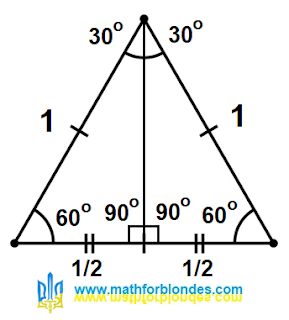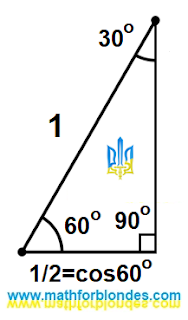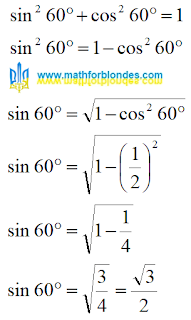## 3/03/2023

### What is the sine of 60 degrees?

Before we look for the answer to the question: "What is the sine of 60 degrees?", I strongly recommend reading the post "Why is the sine of 30 degrees equal to half one?".

## Height of an equilateral triangle

I again take an equilateral triangle with a side equal to one. I draw height.Height of an equilateral triangle

After that, I will not turn anything. I'll just remove half of the drawing.

## Cosine 60 degrees

I have a right-angled triangle, the diagonal of which is equal to one.Cosine 60 degrees

The base of this triangle is equal to 1/2 and is also equal to the cosine of an angle of 60 degrees.

## Sine 60 degrees

The height of this triangle is equal to the sine of the angle of 60 degrees. We calculate the height using the Pythagorean theorem.Sine 60 degrees

The sine of 60 degrees is equal to the square root of three divided by two. It is calculated in the same way as the cosine of 30 degrees.